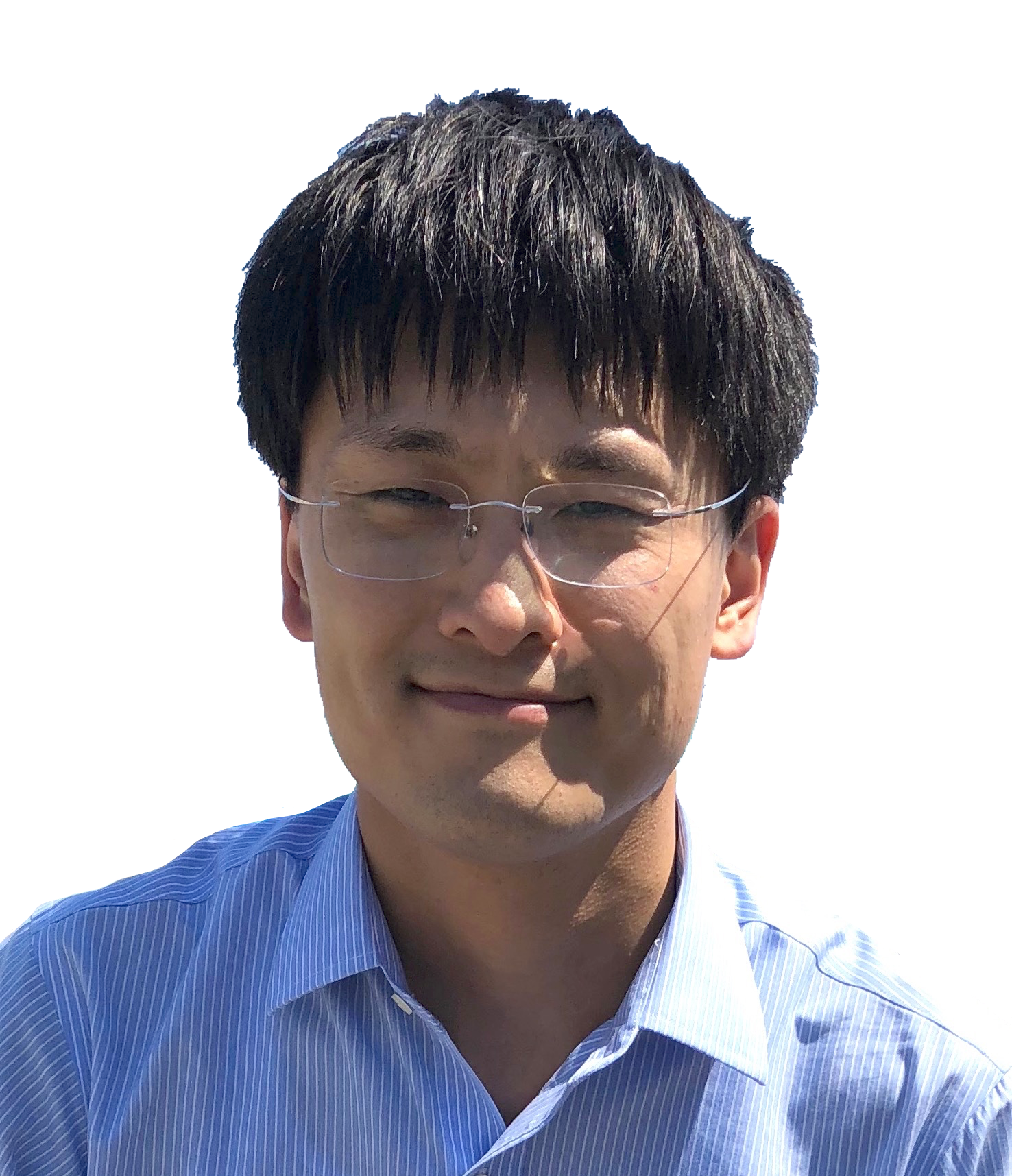DocumentProfessor and RGC Research Fellow
Department of Applied Mathematics
The Hong Kong Polytechnic University
Hung Hom, Hong Kong
https://orcid.org/0000-0001-7566-3464

### Research Interests     Publication     Research Group     PhD and Postdoctoral Positions

• Numerical methods and analysis for partial differential equations, including
• Surface evolution under geometric flows, geometric evolution equations, PDEs on surfaces ← click here
• Low-regularity approximation to nonlinear dispersive equations
• Incompressible Navier–Stokes equations
1. A bounded numerical solution with a small mesh size implies existence of a smooth solution to the Navier–Stokes equations (PDF)
2. A semi-implicit exponential low-regularity integrator for the Navier-Stokes equations (PDF)
3. Analysis of fully discrete finite element methods for 2D Navier-Stokes equations with critical initial data (PDF)
4. Second-order convergence of the linearly extrapolated Crank–Nicolson method for the Navier-Stokes equations with H1 initial data (PDF)
5. A convergent post-processed discontinuous Galerkin method for incompressible flow with variable density (PDF)
• Semilinear parabolic equations and phase field equation
• Interior penalty finite element methods and perfectly matched layer (PML) for the Helmholtz equation
• Maximal Lp-regularity of time discretization methods for parabolic equations
• Maximum-norm stability and maximal Lp-regularity of finite element methods
• High-order approximation of singular solutions of fractional evolution equations
• Dynamic Ginzburg–Landau superconductivity equations in nonsmooth domains
• Time-dependent Joule heating problem (for thermistors with temperature-dependent electric conductivity (PDF)
• ### Editorial boards

• Mathematics of Computation:                 2022.2 –– present

• SIAM Journal on Numerical Analysis:   2022.1 –– present

### Awards

• RGC Research Fellow Award 2023 (by the Hong Kong Research Grants Council).

• The Hong Kong Mathematical Society Young Scholars Award 2022.

• Alexander von Humboldt Research Fellowship, 2015-2016.

• Best Paper Award for Young Scholars 2013, The Chinese Society for Computational Mathematics
(于第十二届全国高校计算数学年会).